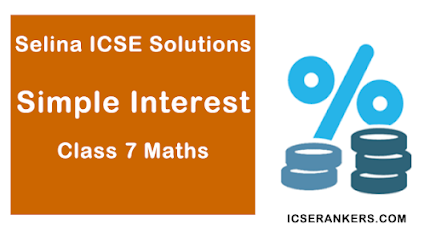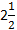# Selina Concise Solutions for Chapter 10 Simple Interest Class 7 ICSE Mathematics

##### Exercise 10

1. Find the S.I. and amount on :

(i) Rs. 150 for 4 years at 5% per year.
(ii) Rs. 350 foryears at 8% p.a.
(iii) Rs. 620 for 4 months at 8 p. per rupee per month.
(iv) Rs. 3,380 for 30 months at% p.a.
(v) 600 from July 12 to Dec. 5 at 10% p.a.
(vi) Rs. 850 from 10th March to 3rd August at% p.a.
(vii) Rs. 225 for 3 years 9 months at 16% p.a.

(i) P = Rs. 150, R = 5% per year ,  T = 4 years

2. On what sum of money does the S.I. for 10 years at 5% become Rs. 1,600 ?
S.I. = Rs. 1600, R = 5% p.a.
T = 10 years

3. Find the time in which Rs. 2,000 will amount to Rs. 2,330 at 11% p.a. ?
Amount (A) = Rs. 2330
Principal (P ) = Rs. 2000
∴ S.I. = A - P = Rs. 2330 - Rs. 2000 = Rs. 330
R = 11% p.a.

4. In what time will a sum of money double it self at 8% p.a ?
Let the principal (P) = ₹ 100
∴ Amount (A) = ₹ 100×2 = ₹ 200
∴ S.I. = A - P = ₹ 200 - ₹ 100 = ₹ 100
Rate(R) = 8% p.a.

5.  In how many years will be ₹870 amount to ₹1,044, the rate of interest being 2% p.a ?
Principal (P) = ₹ 870
Amount (A) = ₹ 1044
∴ S.I. = P - A = ₹ 1044 - ₹ 870 = ₹ 174

6. Find the rate percent if the S.I. on ₹ 275 is 2 years is ₹ 22.
Principal (P) = ₹ 275, S.I. = ₹ 22
Time = 2 years

7. Find the sum which will amount to ₹700 in 5 years at 8% rate p.a.
Amount = ₹ 700, Rate (R) = 8% p.a.
Time(T) = 5 years
Let principal (P) = ₹ 100
∴ Amount (A) = P + S.I.
= ₹ 100 + 40 = ₹140
If amount is ₹ 140, then principal = ₹ 100
and, if amount is Rs. 700, then principal

8. What is the rate of interest, if ₹3,750 amounts to ₹4,650 in 4 years ?
Principal (P) = ₹ 3750
Amount (A) = ₹ 4650
∴ S.I. = A - P = ₹ 4650 - 3750 = ₹ 900
Time (T) = 4 years .

9. In 4 years, ₹6,000 amount to ₹8,000. In what time will ₹525 amount to ₹700 at the same rate ?
In first case, Principal (P) = ₹ 6000
Amount (A) = ₹ 8000
∴ S.I. = A - P = ₹ 8000 - ₹ 6000 = ₹ 2000
Time(T) = 4 years

Let the sum (P) = Rs. 100

11. What sum of money lent out at 5% for 3 years will produce the same interest as Rs. 900 lent out at 4% for 5 years ?
In second case, Principal (P) = Rs. 900
Rate(R) = 4%, Time(T) = 5 years

12. A sum of Rs. 1,780 become Rs. 2,136 in 4 years, Find :
(i) the rate of interest.
(ii) the sum that will become Rs. 810 in 7 years at the same rate of interest ?
(i) In first case, Principal (P) = Rs, 1780
Amount(A) = Rs. 2136
∴ S.I. = A - P = Rs. 2136 - 1780 = Rs. 356
Time (T) = 4 years

(ii) In second case, Let principal (P) = Rs. 100
Rate(R) = 5% p.a.,  Time(T) = 7 years
∴ Amount = P + S.I. = Rs. 100 + 35 = Rs. 135
If amount is Rs. 135, then principal = Rs. 100
and if amount is Rs. 810, then principal

13. A sum amounts to Rs. 2,652 in 6 years at 5% p.a. simple interest. Find :
(i) the sum
(ii) the time in which the same sum will double itself at the same rate of interest.
(i) In first case, Let principal (P) = Rs. 100
Rate(R) = 5% p.a. , Time (T) = 6 years
and, amount = Rs. 100 + Rs. 30 = Rs. 130
If amount is Rs. 130, then principal = Rs. 100
and, if amount is Rs. 2652, then principal
In second case, Let sum (P) = Rs.  100
Amount (A) = Rs. 100× 2 = Rs. 200
S.I. = A - P = Rs. 200 - 100 = Rs. 100
Rate = 5% p.a.

14. P and Q invest Rs. 36,000 and Rs. 25,000 respectively at the same rate of interest per year. If at the end of 4 years, P gets Rs. 3,080 more interest than Q; find the rate of interest.
P's investment (P1) = Rs. 36000
and Q's investment (P2) = Rs. 25000
Period(T) = 4 years, Let rate of interest = x %
Difference in their interest
= Rs. (1440 - 1000)x  = Rs. 440x
But difference  = Rs. 3080
∴ 440x = 3080 ⇒ x 3080/440  ⇒ x = 7%
∴ Rate of interest = 7% p.a.

15. A sum of money is lent for 5 years at R% simple interest per annum. If the interest earned be one-fourth of the money lent, find the value of R.